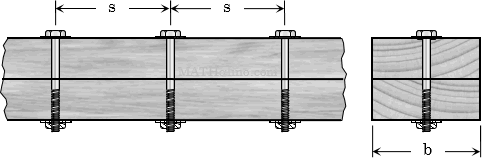# Bolted Connection

Spacing of Bolts / Nails / ScrewsSpacing Formula $s = \dfrac{RI}{VQ}$   or   $s = \dfrac{R}{q}$ Shear Flow Formula $q = \dfrac{VQ}{I}$

Where
s = spacing of bolts
R = shear capacity of bolts
I = moment of inertia of the section about the neutral axis
V = maximum shear
Q = moment of area

Rate: